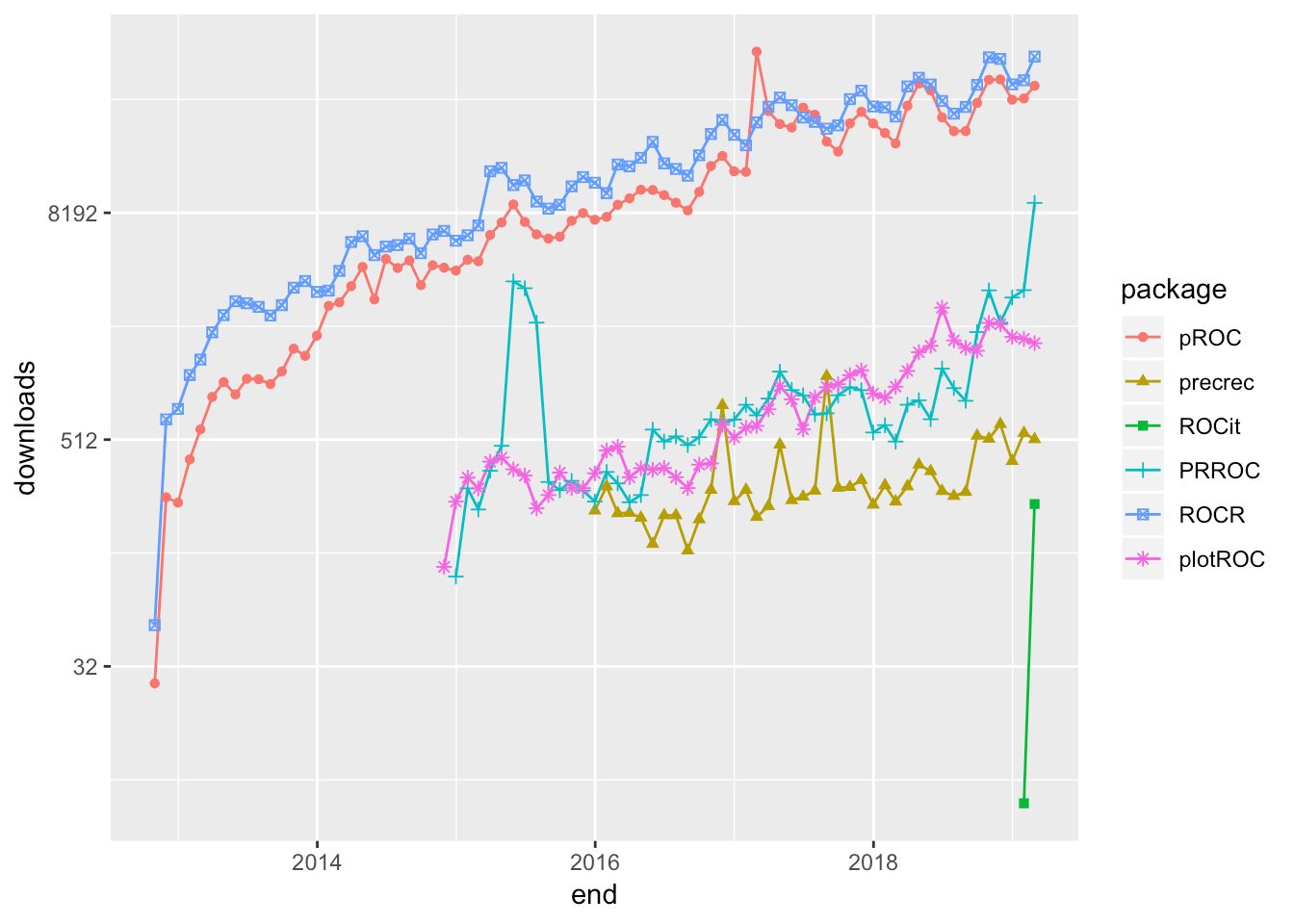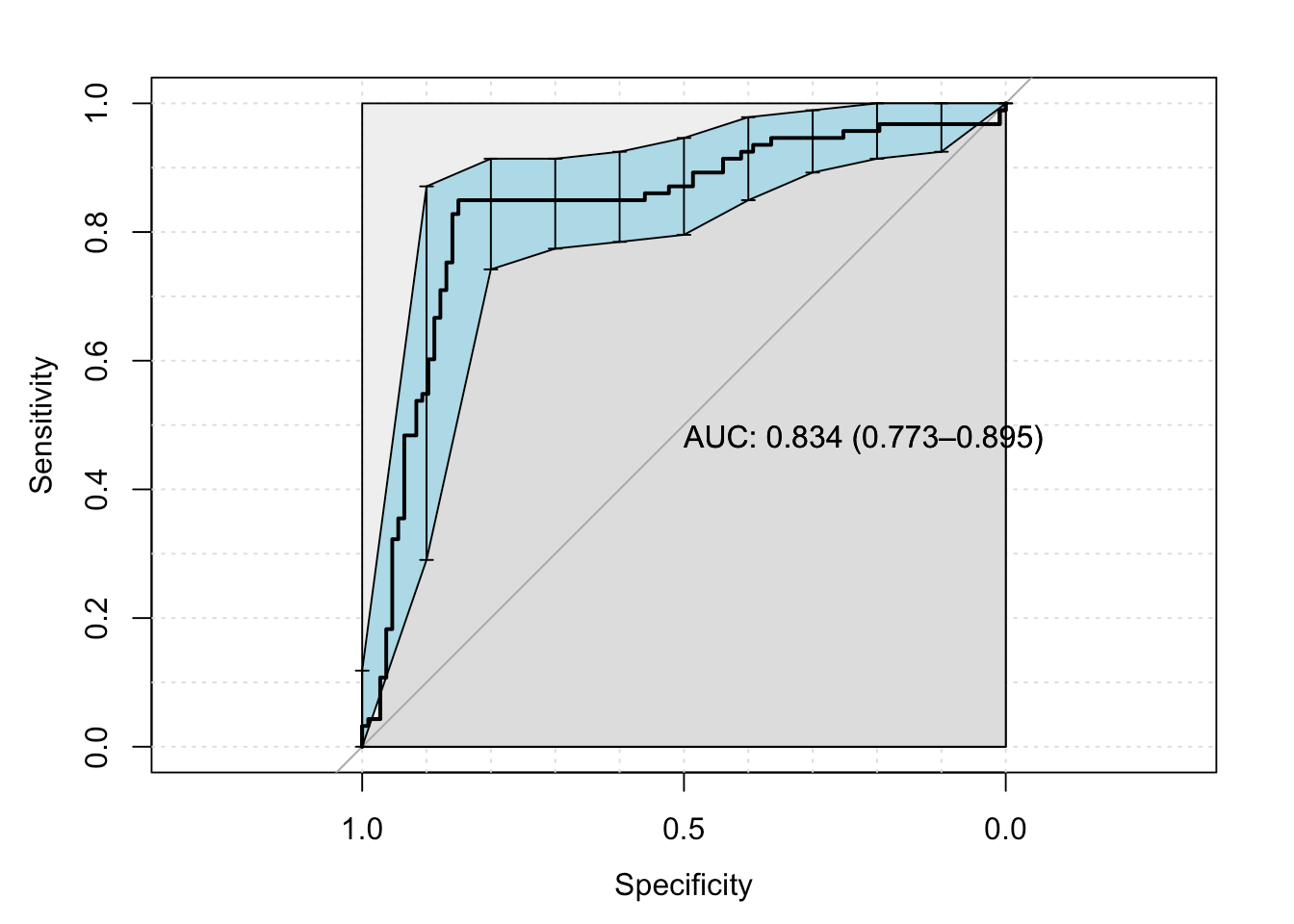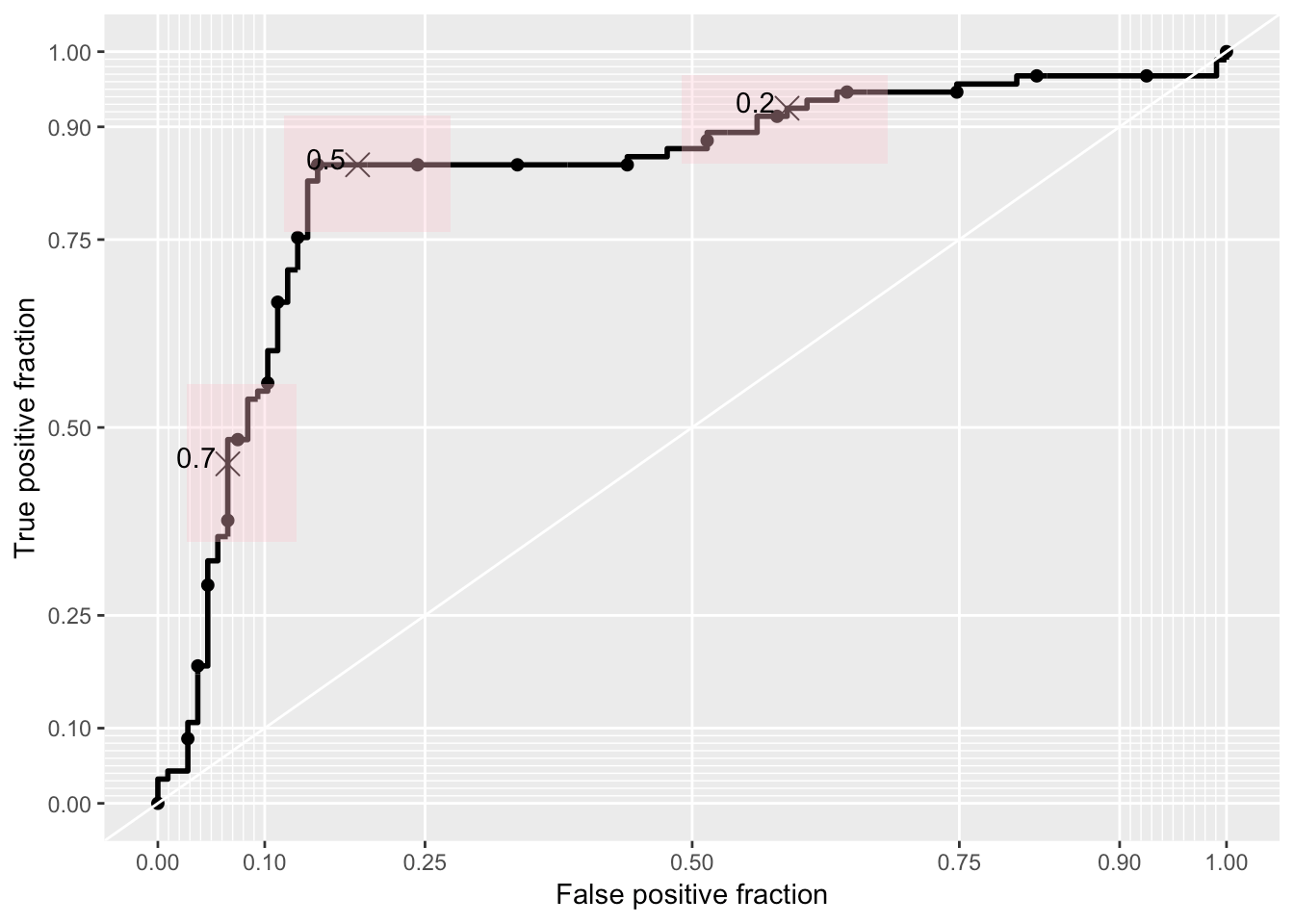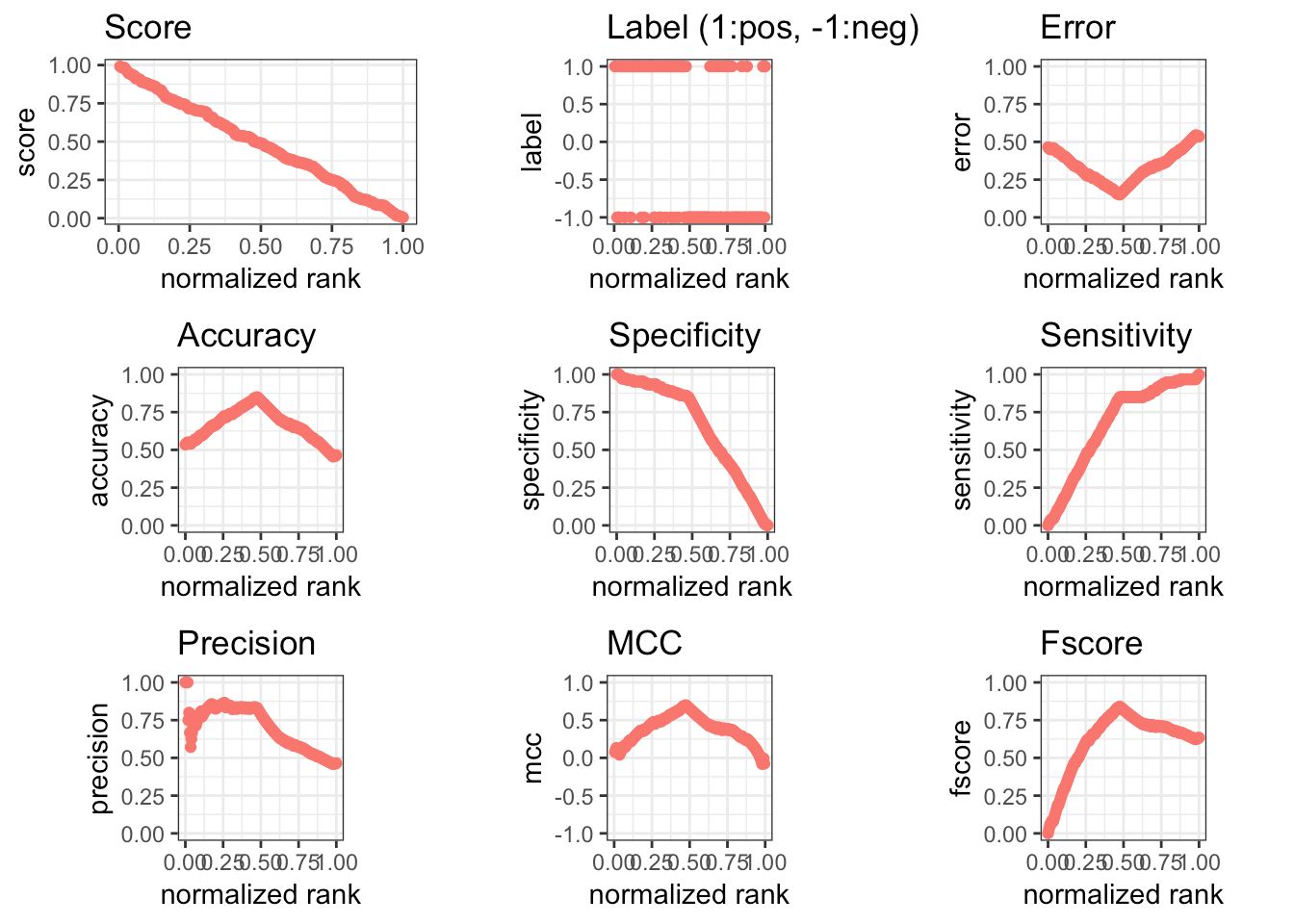Want to share your content on R-bloggers? click here if you have a blog, or here if you don't.

In a recent post, I presented some of the theory underlying ROC curves, and outlined the history leading up to their present popularity for characterizing the performance of machine learning models. In this post, I describe how to search CRAN for packages to plot ROC curves, and highlight six useful packages.

Although I began with a few ideas about packages that I wanted to talk about, like ROCR and pROC, which I have found useful in the past, I decided to use Gábor Csárdi’s relatively new package pkgsearch to search through CRAN and see what’s out there. The `package_search()` function takes a text string as input and uses basic text mining techniques to search all of CRAN. The algorithm searches through package text fields, and produces a score for each package it finds that is weighted by the number of reverse dependencies and downloads.

``````library(tidyverse)  # for data manipulation
library(pkgsearch)  # for searching packages``````

After some trial and error, I settled on the following query, which includes a number of interesting ROC-related packages.

``rocPkg <-  pkg_search(query="ROC",size=200)``

Then, I narrowed down the field to 46 packages by filtering out orphaned packages and packages with a score less than 190.

``````rocPkgShort <- rocPkg %>%
filter(maintainer_name != "ORPHANED", score > 190) %>%
``````## # A tibble: 6 x 3
##
## 1  690. ROCR                    56356
## 2 7938. pROC                    39584
## 3 1328. PRROC                    9058
## 4  833. sROC                     4236
## 5  266. hmeasure                 1946
## 6 1021. plotROC                  1672``````

To complete the selection process, I did the hard work of browsing the documentation for the packages to pick out what I thought would be generally useful to most data scientists. The following plot uses Guangchuang Yu’s `dlstats` package to look at the download history for the six packages I selected to profile.

``````library(dlstats)
shortList <- c("pROC","precrec","ROCit", "PRROC","ROCR","plotROC")
geom_line() + geom_point(aes(shape=package)) +
scale_y_continuous(trans = 'log2')``````### ROCR – 2005

ROCR has been around for almost 14 years, and has be a rock-solid workhorse for drawing ROC curves. I particularly like the way the `performance()` function has you set up calculation of the curve by entering the true positive rate, `tpr`, and false positive rate, `fpr`, parameters. Not only is this reassuringly transparent, it shows the flexibility to calculate nearly every performance measure for a binary classifier by entering the appropriate parameter. For example, to produce a precision-recall curve, you would enter `prec` and `rec`. Although there is no vignette, the documentation of the package is very good.

The following code sets up and plots the default `ROCR` ROC curve using a synthetic data set that comes with the package. I will use this same data set throughout this post.

``library(ROCR)``
``## Loading required package: gplots``
``````##
## Attaching package: 'gplots'``````
``````## The following object is masked from 'package:stats':
##
##     lowess``````
``````# plot a ROC curve for a single prediction run
# and color the curve according to cutoff.
data(ROCR.simple)
df <- data.frame(ROCR.simple)
pred <- prediction(df\$predictions, df\$labels)
perf <- performance(pred,"tpr","fpr")
plot(perf,colorize=TRUE)``````### pROC – 2010

It is clear from the downloads curve that `pROC` is also popular with data scientists. I like that it is pretty easy to get confidence intervals for the Area Under the Curve, `AUC`, on the plot.

``library(pROC)``
``## Type 'citation("pROC")' for a citation.``
``````##
## Attaching package: 'pROC'``````
``````## The following objects are masked from 'package:stats':
##
##     cov, smooth, var``````
``````pROC_obj <- roc(df\$labels,df\$predictions,
smoothed = TRUE,
# arguments for ci
ci=TRUE, ci.alpha=0.9, stratified=FALSE,
# arguments for plot
plot=TRUE, auc.polygon=TRUE, max.auc.polygon=TRUE, grid=TRUE,
print.auc=TRUE, show.thres=TRUE)

sens.ci <- ci.se(pROC_obj)
plot(sens.ci, type="shape", col="lightblue")``````
``````## Warning in plot.ci.se(sens.ci, type = "shape", col = "lightblue"): Low
## definition shape.``````
``plot(sens.ci, type="bars")``### PRROC – 2014

Although not nearly as popular as `ROCR` and `pROC`, `PRROC` seems to be making a bit of a comeback lately. The terminology for the inputs is a bit eclectic, but once you figure that out the `roc.curve()` function plots a clean ROC curve with minimal fuss. `PRROC` is really set up to do precision-recall curves as the vignette indicates.

``````library(PRROC)

PRROC_obj <- roc.curve(scores.class0 = df\$predictions, weights.class0=df\$labels,
curve=TRUE)
plot(PRROC_obj)``````### plotROC – 2014

`plotROC` is an excellent choice for drawing ROC curves with `ggplot()`. My guess is that it appears to enjoy only limited popularity because the documentation uses medical terminology like “disease status” and “markers”. Nevertheless, the documentation, which includes both a vignette and a Shiny application, is very good.

The package offers a number of feature-rich `ggplot()` geoms that enable the production of elaborate plots. The following plot contains some styling, and includes Clopper and Pearson (1934) exact method confidence intervals.

``````library(plotROC)
rocplot <- ggplot(df, aes(m = predictions, d = labels))+ geom_roc(n.cuts=20,labels=FALSE)
rocplot + style_roc(theme = theme_grey) + geom_rocci(fill="pink") ``````### precrec – 2015

`precrec` is another library for plotting ROC and precision-recall curves.

``library(precrec)``
``````##
## Attaching package: 'precrec'``````
``````## The following object is masked from 'package:pROC':
##
##     auc``````
``````precrec_obj <- evalmod(scores = df\$predictions, labels = df\$labels)
autoplot(precrec_obj)``````Parameter options for the `evalmod()` function make it easy to produce basic plots of various model features.

``````precrec_obj2 <- evalmod(scores = df\$predictions, labels = df\$labels, mode="basic")
autoplot(precrec_obj2)   ``````### ROCit – 2019

`ROCit` is a new package for plotting ROC curves and other binary classification visualizations that rocketed onto the scene in January, and is climbing quickly in popularity. I would never have discovered it if I had automatically filtered my original search by downloads. The default plot includes the location of the Yourden’s J Statistic.

``library(ROCit)``
``## Warning: package 'ROCit' was built under R version 3.5.2``
``````ROCit_obj <- rocit(score=df\$predictions,class=df\$labels)
plot(ROCit_obj)``````Several other visualizations are possible. The following plot shows the cumulative densities of the positive and negative responses. The KS statistic shows the maximum distance between the two curves.

``ksplot(ROCit_obj)``In this attempt to dig into CRAN and uncover some of the resources R contains for plotting ROC curves and other binary classifier visualizations, I have only scratched the surface. Moreover, I have deliberately ignored the many packages available for specialized applications, such as survivalROC for computing time-dependent ROC curves from censored survival data, and cvAUC, which contains functions for evaluating cross-validated AUC measures. Nevertheless, I hope that this little exercise will help you find what you are looking for.